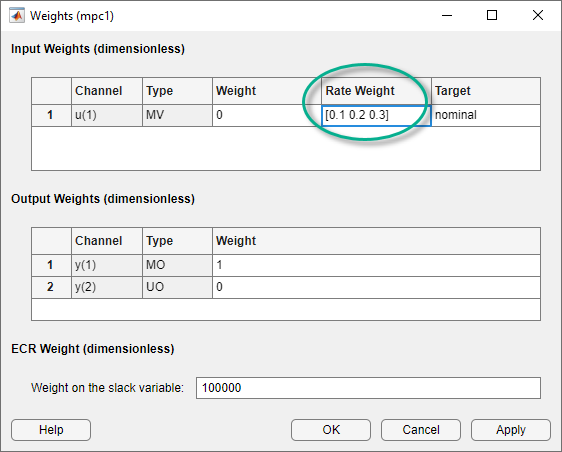Main Content

## Time-Varying Weights and Constraints

### Time-Varying Weights

As explained in Optimization Problem, the wy, wu, and w∆u weights can change from one step in the prediction horizon to the next. Such a time-varying weight is an array containing p rows, where p is the prediction horizon, and either ny or nu columns (number of OVs or MVs).

Using time-varying weights provides additional tuning possibilities. However, it complicates tuning. Recommended practice is to use constant weights unless your application includes unusual characteristics. For example, an application requiring terminal weights must employ time-varying weights. See Terminal Weights and Constraints.

You can specify time-varying weights in MPC Designer. In the Weights dialog box, specify a time-varying weight as a vector. Each element of the vector corresponds to one step in the prediction horizon. If the length of the vector is less than p, the last weight value applies for the remainder of the prediction horizon.Note

For any given input channel, you can specify different vector lengths for Rate Weight and Weight. However, if you specify a time-varying Weight for any input channel, you must specify a time-varying Weight for all inputs using the same length weight vectors. Similarly, all input Rate Weight values must use the same vector length.

Also, if you specify a time-varying Weight for any output channel, you must specify a time-varying Weight for all output using the same length weight vectors.

### Time-Varying Constraints

When bounding an MV, OV, or MV increment, you can use a different bound value at each prediction-horizon step. To do so, specify the bound as a vector of up to p values, where p is the prediction horizon length (number of control intervals). If you specify n < p values, the nth value applies for the remaining pn steps.

You can remove constraints at selected steps by specifying `Inf` (or `-Inf`).

If plant delays prevent the MVs from affecting an OV during the first d steps of the prediction horizon and you must include bounds on that OV, leave the OV unconstrained for the first d steps.

You can specify time-varying constraints in MPC Designer. In the Constraints dialog box, specify a vector for each time-varying constraint.## Support

#### Implementing an Adaptive Cruise Controller with Simulink

Download technical paper